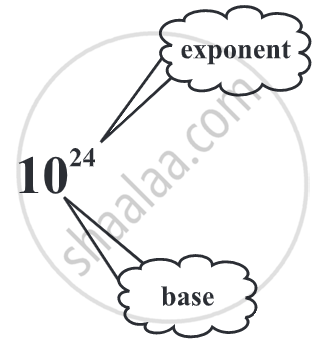# Concept of Exponents

## Definition

Exponents: An exponent is a numerical notation that indicates the number of times a number is to be multiplied by itself. Exponent is also called as power or index. Example, 75.

## Notes

### Introduction of Exponents and powers:

Distance between Sun and Saturn is 1,433,500,000,000 m and distance between Saturn and Uranus is 1,439,000,000,000 m.

We can’t read such numbers. These very large numbers are difficult to read, understand, and compare. To make these numbers easy to read, understand, and compare, we use exponents.

#### 1. Exponents:

• An exponent is a numerical notation that indicates the number of times a number is to be multiplied by itself.

• Exponent is also called as power or index.

• The main purpose of learning exponents is to write a very big number or a larger number in the simplest form.

• 10, 000 = 10 × 10 × 10 × 10 = 104.
The short notation 104 stands for the product 10 × 10 × 10 × 10.
104 is called the exponential form of 10,000.• Generally, exponent says that how many times the base should be multiplied.

If 75 is given the meaning is 7 is multiplied 5 times i.e. 7 × 7 × 7 × 7 × 7.

• Here ‘7’ is called the base and ‘5’ the exponent.

• The number 75 is read as 7 raised to the power of 5 or simply as the fifth power of 7.

• Generally, if a is any number, a × a × a ×.......... (m times) = am
Read am as ‘a raised to the power m’ or ‘the mth power of a’.
Here m is a natural number.
∴ 54 = 5 × 5 × 5 × 5 = 625. Or, the value of the number 54 = 625.

#### Base with negative integer:

• (– 10)3 = (– 10) × (– 10) × (– 10) = 100 × (– 10) = – 1000
So, (–1)odd number = - 1

• (–5)4 = (– 5) × (– 5) × (– 5) × (– 5) = 25 × 25 = 625
So, (–1)even number = + 1

#### 2. Comparison of quantities using exponents:

• If two numbers in standard form have the same power of 10, then the number with the larger factor is greater.
For example, 8.72 × 1024 < 9.4326 × 1024.

• If two numbers in standard form have the same factor, then the number with the larger power of 10 will be greater.
For example, 8.72 × 108 > 8.72 × 104.

• If two numbers in standard form have different factors and the different power of 10, then the number with the higher power of ten (i.e., the larger exponent) is the larger number.
For example, 8.72 × 1024 > 9.4326 × 1023.

## Example

Which one is greater 23 or 32?

We have, 23 = 2 × 2 × 2 = 8 and 32 = 3 × 3 = 9.

Since 9 > 8,
so, 32 is greater than 23.

## Example

Expand a3b2, a2b3, b2a3, b3a2. Are they all same?

a3b2 = a3 × b2 = (a × a × a) × (b × b) = a × a × a × b × b

a2b3 = a2 × b3 = a × a × b × b × b

b2a3 = b2 × a3 = b × b × a × a × a

b3a2 = b3 × a2 = b × b × b × a × a

a3b2 and a2b3 the powers of a and b are different. Thus a3b2 and a2b3 are different.

a3b2 and b2a3 are the same, since the powers of a and b in thesetwo terms are the same. The order of factors does not matter.

Thus, a3b2 = a3 × b2 = b2 × a3 = b2a3. Similarly, a2b3 and b3a2 are the same.

## Example

Express the following number as a product of powers of prime factor: 432

432

= 2 × 216 = 2 × 2 × 108

= 2 × 2 × 2 × 54

= 2 × 2 × 2 × 2 × 27

= 2 × 2 × 2 × 2 × 3 × 9

= 2 × 2 × 2 × 2 × 3 × 3 × 3

or 432 = 24 × 33.

## Example

Express the following number as a product of powers of prime factor: 16000

16,000

= 16 × 1000

= (2 × 2 × 2 × 2) × 1000

= 24 × 103 .......(as 16 = 2 × 2 × 2 × 2)

= (2 × 2 × 2 × 2) × (2 × 2 × 2 × 5 × 5 × 5) = 24 × 23 × 53.......(Since, 1000 = 2 × 2 × 2 × 5 × 5 × 5)

= (2 × 2 × 2 × 2 × 2 × 2 × 2 ) × (5 × 5 × 5)

or, 16,000 = 27 × 53.

## Example

Work out (1)5, (–1)3, (–1)4, (–10)3, (–5)4

i) (1)5 = 1 × 1 × 1 × 1 × 1 = 1

ii) (–1)3 = (– 1) × (– 1) × (– 1) = 1 × (– 1) = – 1

iii) (–1)4 = (–1) × (–1) × (–1) × (–1) = 1 ×1 = 1

iv) (–10)3 = (–10) × (–10) × (–10) = 100 × (– 10) = – 1000

v) (–5)4 = (–5) × (–5) × (–5) × (–5) = 25 × 25 = 625

If you would like to contribute notes or other learning material, please submit them using the button below.

### Shaalaa.com

Introduction of Exponents & Powers [00:12:20]
S
0%